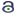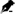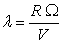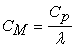﻿ Experimental Investigation of the Reynolds Number’S Effect on the Aerodynamic Characteristics of a Horizontal Axis Wind Turbine of the Göttingen 188 Airfoil Type

### Experimental Investigation of the Reynolds Number’S Effect on the Aerodynamic Characteristics of a H...

Zied Driss, Sarhan Karray, Ali Damak, Mohamed Salah AbidOPEN ACCESSPEER-REVIEWED

## Experimental Investigation of the Reynolds Number’S Effect on the Aerodynamic Characteristics of a Horizontal Axis Wind Turbine of the Göttingen 188 Airfoil Type

Zied Driss1,, Sarhan Karray1, Ali Damak1, Mohamed Salah Abid1

1Laboratory of Electro-Mechanic Systems (LASEM), National School of Engineers of Sfax (ENIS), University of Sfax (US), Tunisia

### Abstract

In this paper, a study of the effect of Reynolds number on the aerodynamic characteristics of a horizontal axis wind turbine equipped by three adjustable blades of the Göttingen 188 airfoil has been developed. Particularly, different aerodynamic regimes defined by Reynolds numbers were investigated. To achieve this, an open wind tunnel has been used to determine the global characteristics of the wind turbine. The obtained results consist of the recovered power, the exerted torque on the rotor in static and in dynamic modes as well as power coefficient and torque coefficient. This work has been developed at Laboratory of Electro-Mechanic Systems (LASEM) of the National School of Engineers of Sfax (ENIS).

### At a glance: Figures

123
Prev Next

• Driss, Zied, et al. "Experimental Investigation of the Reynolds Number’S Effect on the Aerodynamic Characteristics of a Horizontal Axis Wind Turbine of the Göttingen 188 Airfoil Type." American Journal of Mechanical Engineering 1.5 (2013): 143-148.
• Driss, Z. , Karray, S. , Damak, A. , & Abid, M. S. (2013). Experimental Investigation of the Reynolds Number’S Effect on the Aerodynamic Characteristics of a Horizontal Axis Wind Turbine of the Göttingen 188 Airfoil Type. American Journal of Mechanical Engineering, 1(5), 143-148.
• Driss, Zied, Sarhan Karray, Ali Damak, and Mohamed Salah Abid. "Experimental Investigation of the Reynolds Number’S Effect on the Aerodynamic Characteristics of a Horizontal Axis Wind Turbine of the Göttingen 188 Airfoil Type." American Journal of Mechanical Engineering 1, no. 5 (2013): 143-148.

 Import into BibTeX Import into EndNote Import into RefMan Import into RefWorks

### 1. Introduction

On the basis of the previous studies, it appears that there is paucity on the study of small horizontal axis wind turbine and particularly on the Göttingen airfoil type. For this reason, an experimental investigation is presented in this paper to study the effect of the Reynolds number on the aerodynamic characteristics of a horizontal axis wind turbine equipped by three adjustable blades of the Göttingen 188 airfoil.

### 2. Geometrical Arrangement

The present work focuses on the horizontal axis wind turbine. The wind turbine is constituted of three adjustable blades of the Göttingen 188 airfoil. In this application, the airfoil is characterised by a blade length equal to l = 100 mm and a chord length equal to C = 43 mm. The radius rotor is equal to R = 157 mm (Figure 1). Indeed, the wind turbine is equipped by a system to change the wedging angle β, measured between the blade rotation plane and the chorde. The experimental investigation has been developed using wind tunnel. The wind turbine has been introduced through a hole situated on the top of the test vein. Particularly, a vertical axis is used to maintain the rotor. This installation permits to study the effect of the wedging angle and the Reynolds number on the global characteristics of the wind turbine (Figure 2).

Figure 1. Horizontal axis wind turbine equipped by three adjustable blades of the Göttingen 188 airfoil

### 3. Experimental Method

In this study, an experimental investigation is conducted on an open wind tunnel designed and realized in our Laboratory of Electro-Mechanic Systems at National School of Engineers of Sfax [7, 8, 9, 10]. To do this, the necessary equipments for the global characterization of the horizontal axis wind turbines have been installed. This involved the manufacture of an open wind tunnel and its instrumentation. This system has been designed and realised to establish the aerodynamic characteristics of small wind turbines. It mainly consists of five compartments: a settling chamber, a collector, a test vein, a diffuser and a drive section. A vacuum cleaner with variable speed draws the air through the test vein. The honeycomb placed at the input of this room provides a uniform airflow. The hot wire anemometry technique has been used to justify the nature of the flow generated by the system and to ensure a uniform airflow in the test vein. The experimental device has been used to predict the aerodynamic behaviour and investigate the conditions experienced by the wind turbines placed in the air flow. The rotor axis has been placed in the middle of the test vein having a cross section area of 400 mm x 400 mm. By changing the rotation frequency of the vacuum cleaner SV0081C5-1F type, the wind tunnel exit-air velocity was controlled. The entire tests have been conducted within a hot wire anemometry AM-4204 model to measure the air velocity. In the test vein, the maximum air velocity value is equal to 12.7 m/s. The rotational speed of the wind turbine rotor was measured with a digital tachometer CA-27 model. To measure the static torque on the rotor shaft, a torque meter TQ-8800 model has been used. The dynamic torque exerted on the rotor shaft was measured with a DC generator which transforms the torque on its axis at an electrical current. For that the generator, coupled to the dynamometer RZR-2102 model, display simultaneously the shape speed and the dynamic torque. This dynamometer has been used to provide mechanical power to the generator which delivers an electric current in a resistive load. Torque measurement integrated into the dynamometer, allows tracing the calibration curve that connects the electric current supplied by the generator to the dynamic torque (Figure 3). This calibration curve serves for determination of the dynamic torque after referring to the value of the electric current supplied by the generator. This strategy offered a comprehensive understanding of aerodynamic characteristics of different configurations.

### 4. Experimental Results

In this study, different flow regimes defined by the Reynolds numbers equals to Re = 165093, Re = 194350, Re = 217338, Re = 242415, Re = 257044 and Re = 265403 are investigated.

4.1. Power

Figure 4 presents the variation of the recovered power depending on the revolution speed Ω of the horizontal axis-wind turbine for different Reynolds numbers equals to Re = 165093, Re = 194350, Re = 217338, Re = 242415, Re = 257044 and Re = 265403. According to these results, the presented curve shows a parabolic branch. The recovered power decreases with the increase of the revolution speed. Indeed, it’s noted that the Reynolds number has a direct effect on the results. In these conditions, the recovered power increases with the increase of the Reynolds number. The maximal values of the recovered power increase also with the increase of the Reynolds number. Particularly, for the Reynolds number Re = 165093, the maximal value of the recovered power is equal to P = 8.4 W for a revolution speed equal to Ω = 885 rpm. Indeed, the minimal and maximal revolution speeds increase with the increase of the Reynolds number. In fact, for a Reynolds number Re = 165093, the revolution speed of the wind turbine varies between Ω = 885 rpm and Ω = 1090 rpm. This interval variation increases for a Reynolds number equal to Re = 265403. In this case, the revolution speed varies between Ω = 2010 rpm and Ω = 2100 rpm.

4.2. Power Coefficient

Figure 5 presents the variation of the power coefficient depending on the specific velocity λ of the horizontal axis-wind turbine for different Reynolds numbers equal to Re = 165093, Re = 194350, Re = 217338, Re = 242415, Re = 257044 and Re = 265403. According to these results, the presented curve shows a parabolic branch. Indeed, it’s noted that the Reynolds number has a direct effect on the results. Particularly, the power coefficient increases with the increase of the Reynolds number at the same specific velocity λ. In these conditions, for the Reynolds number Re = 165093, the maximal value of the power coefficient is equal to Cp = 0.21 for a specific velocity equal to λ = 2. Indeed, the minimal and maximal specific velocity values increase with the increase of the Reynolds number. In fact, for a Reynolds number Re = 165093, the specific velocity of the wind turbine varies between λ = 2 and λ = 2.3. However, with a Reynolds number Re = 265403, the specific velocity varies between λ = 2.63 and λ = 2.72.

4.3. Dynamic Torque

Figure 6 presents the variation of the dynamic torque depending on the revolution speed Ω of the horizontal axis-wind turbine for different Reynolds numbers equal to Re = 165093, Re = 194350, Re = 217338, Re = 242415, Re = 257044 and Re = 265403. According to these results, the dynamic torque value decreases with the increase of the revolution speed Ω. Also, it’s clear that the Reynolds number has a direct effect on the cartographies presentation. Particularly, the dynamic torque value increases with the increase of the Reynolds number at the same revolution speed Ω. In fact, the maximal value of the dynamic torque reaches Md = 0,072 N.m for the Reynolds number equal to Re = 165093 and a revolution speed equal to Ω = 925 rpm. However, the maximal value of the dynamic torque reaches Md = 0,088 N.m for the Reynolds number equal to Re = 265403 and a revolution speed equal to Ω = 2002 rpm. This confirms that the maximal value increases with the increase of the Reynolds number. Indeed, it’s clear that the minimal value of the dynamic torque increases with the increase of the Reynolds number. For example, the minimal value of the dynamic torque reaches Md = 0,008 N.m for the Reynolds number equal to Re = 265403 and a revolution speed equal to Ω = 2140 rpm.

4.4. Dynamic Torque Coefficient

Figure 7 presents the variation of the dynamic torque coefficient depending on the specific velocity λ of the horizontal axis-wind turbine for different Reynolds numbers equal to Re = 165093, Re = 194350, Re = 217338, Re = 242415, Re = 257044 and Re = 265403. According to these results, it’s noted that the presented curve shows a parabolic branch. In these conditions, the dynamic torque coefficient decreases with the increase of the specific velocity λ. Indeed, it’s clear that the Reynolds number has a direct effect on the cartographies presentation. The maximal values of the dynamic torque coefficient decrease also with the increase of the Reynolds number. In fact, for the Reynolds number Re = 165093, the maximal value of the dynamic torque coefficient is equal to CMd = 0.108 for a specific velocity equal to λ = 1.94. However, for the Reynolds number Re = 265403, the maximal value of the dynamic torque coefficient is equal to CMd = 0.059 for a specific velocity equal to λ = 2.52.

4.5. Static Torque

Figure 8 presents the variation of the static torque depending on the wedging angle β of the horizontal axis-wind turbine for different Reynolds numbers equals to Re = 165093, Re = 194350, Re = 217338, Re = 242415, Re = 257044 and Re = 265403. According to these results, the presented curve shows a parabolic branch. Indeed, it’s noted that the Reynolds number has a direct effect on the cartographies presentation.

In these conditions, the static torque increases with the increase of the Reynolds number. Particularly, the maximal values of the static torque increase with the increase of the Reynolds number. In fact, for the Reynolds number Re = 165093, the maximal value of the static torque is equal to Ms = 2.8 N.m for a wedging angle equal to β = 50°.

Figure 9. Variation of the static torque coefficient depending on the wedging angle β
4.6. Static Torque Coefficient

Figure 9 presents the variation of the static torque coefficient depending on the wedging angle β of the horizontal axis-wind turbine for different Reynolds numbers equal to Re = 165093, Re = 194350, Re = 217338, Re = 242415, Re = 257044 and Re = 265403. According to these results, it’s noted that the presented curve shows a parabolic branch. Indeed, it’s clear that the Reynolds number hasn’t an effect on the cartographies presentation. In these conditions, the maximal value of the static torque coefficient value is equal to CMs = 0.06 for a wedging angle equal to β = 50°. Indeed, the static torque coefficient value becomes null for a wedging angle equal to β = 0°.

### 5. Conclusion

In this paper, an experimental investigation has been developed to study the Reynolds numbers effect on the global characteristics of the horizontal axis wind turbine equipped by three adjustable blades of the Göttingen 188 airfoil. Evaluation of the rotor performance based on the power and torque produced is reported to optimize and to improve the experimental conditions of the wind turbine. According to the experimental results, it’s noted that the Reynolds number has a direct effect on the global characteristics, except the static torque coefficient.

In the future, we intend to change the blade profiles to optimize the output of the wind turbines. Therefore, we propose to develop an experimental investigation within a particle image velocimetry laser (PIV) system for a finer survey of the local out-flow features. Also, it is interested to find the necessary material for the manufacture of the wind turbines.

### Nomenclature

A: Swept area of the rotor (m2)

C: chord length (m)

D: diameter of the rotor (m)

l: length of the blade (m)

M: Torque (N.m)

P: power (W)

R: radius of the rotor (m)

V: Speed of air (m/s)

### Greek Letters

β: wedging angle (°)

μ: dynamic viscosity of the fluide (Pa.s)

Ω: revolution speed of the rotor (rpm)

ρ: density of fluid (kg.m-3): Reynolds number: Specific velocity: Coefficient of power: Coefficient of torque

s: static

d: dynamic

### References

  D. HU, Near wake of a model horizontal-axis wind turbine, Journal of Hydrodynamics 21 (2) (2009) 285-291.In article CrossRef  I. Grant, M. Mo, X. Pan, P. Parkin, J. Powell, H. Reinecke, K. Shuang, F. Coton, D. Lee, An experimental and numerical study of the vortex laments in the wake of an operational, horizontal-axis, wind turbine, Journal of Wind Engineering and Industrial Aerodynamics 85 (2000) 177-189.In article CrossRef  M.J. Barnsley, J.F. Wellicome, Wind tunnel investigation of stall aerodynamics for a 1.0 m horizontal axis rotor, Journal of Wind Engineering and Industrial Aerodynamics 39 (1992) 11-21.In article CrossRef  C.C. Ting, C.W. Lai, C.B. Huang, Developing the dual system of wind chiller integrated with wind generator, Applied Energy 88 (2011) 741-747.In article CrossRef  Z. Driss, W. Triki, M. S. Abid, Numerical investigation of the Rutland 913 wind turbine airfoils effect on the aerodynamic structure flow, Science Academy Transactions on Renewable Energy Systems Engineering and Technology 1 (4) (2011) 116-123.In article  T.Y. Chen, L.R. Liou, Blockage corrections in wind tunnel tests of small horizontal-axis wind turbines, Experimental Thermal and Fluid Science 35 (2011) 565-569.In article CrossRef  Z. Driss, M. S. Abid, Numerical and experimental study of an open circuit tunnel: aerodynamic characteristics, Science Academy Transactions on Renewable Energy Systems Engineering and Technology 2 (1) (2012) 116-123.In article  Z. Driss, A. Damak, H. Kchaou, M.S. Abid, Experimental investigation on wind tunnel, Tunisian Japanese Symposium on Science, Society and (2011) 1-4.In article  A. Damak, Z. Driss, H. Kchaou, M.S. Abid, Conception et réalisation d’une soufflerie à aspiration, 4ème Congrès International Conception et Modélisation des Systèmes Mécaniques (2011) 1-7.In article  A. Damak, Z. Driss, M.S. Abid, Experimental investigation of helical Savonius rotor with a twist of 180°, Renewable Energy 52 (2013) 136-142.In article CrossRef